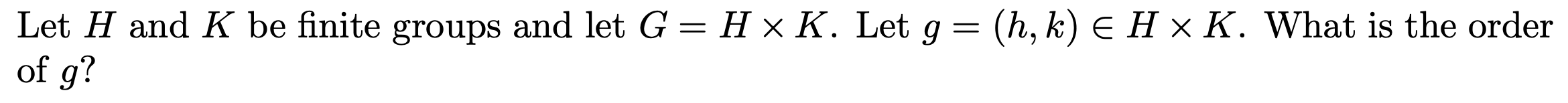(h, k) E H x K. What is the orderLet H and K be finite groups and let G = H x K. Let gof g?

Questionhelp_outlineImage Transcriptionclose(h, k) E H x K. What is the order Let H and K be finite groups and let G = H x K. Let g of g? fullscreen
Step 1

To calculate the order of the element (h,...

Want to see the full answer?

See Solution

Want to see this answer and more?

Our solutions are written by experts, many with advanced degrees, and available 24/7

See Solution
Tagged in

Math